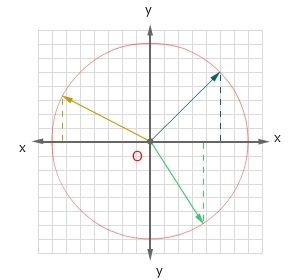# [Flash]Flash与3D编程探秘（四）- 摄像机旋转基础知识#### 关于三角函数object.x = origin.x + Math.cos(angle) * radius;
object.y = origin.y + Math.sin(angle) * radius;

randians = degrees * (Math.PI / 180); PI = 3.1415926535897932384626433832795…

#### 反三角函数

angle = Math.atan2(object.y, object.x);
angle
= Math.atan(y/x);

hypotenuse = Math.sqrt(x*+ y*y);

#### 横向旋转摄像机

= distance * Math.cos(a);
= distance * Math.sin(a);

= x0 + distance * Math.cos(a);
= y0 + distance * Math.sin(a);作者：Yang Zhou 出处：http://yangzhou1030.cnblogs.com 感谢：Yunqing 本文版权归作者和博客园共有，转载未经作者同意必须保留此段声明。请在文章页面明显位置给出原文连接，作者保留追究法律责任的权利。

### 觉得文章有用就打赏一下文章作者

#### 支付宝扫一扫打赏#### 微信扫一扫打赏• QQ咨询
• 回顶